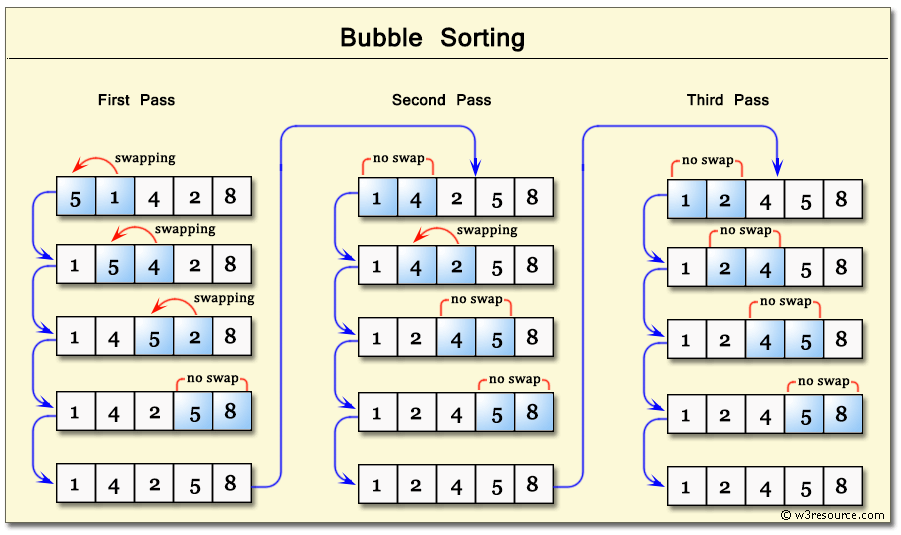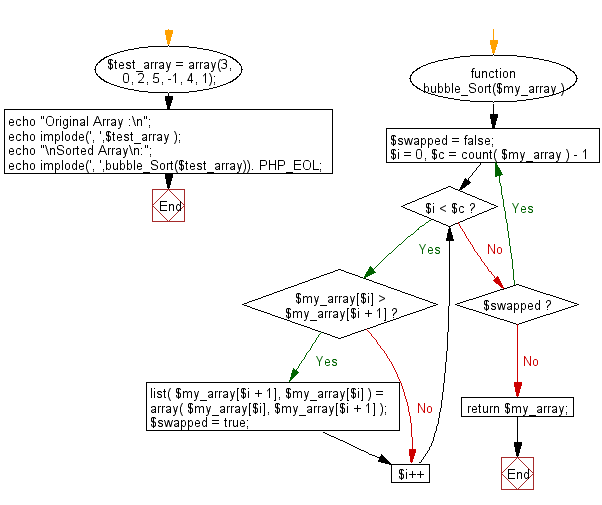﻿ PHP: Sort a list of elements using Bubble sort - w3resource

# PHP Searching and Sorting Algorithm: Bubble sort

## PHP Searching and Sorting Algorithm: Exercise-6 with Solution

Write a PHP program to sort a list of elements using Bubble sort.

According to Wikipedia "Bubble sort, sometimes referred to as sinking sort, is a simple sorting algorithm that repeatedly steps through the list to be sorted, compares each pair of adjacent items and swaps them if they are in the wrong order. The pass through the list is repeated until no swaps are needed, which indicates that the list is sorted. The algorithm, which is a comparison sort, is named for the way smaller elements "bubble" to the top of the list. Although the algorithm is simple, it is too slow and impractical for most problems even when compared to insertion sort. It can be practical if the input is usually in sort order but may occasionally have some out-of-order elements nearly in position."

Step by step pictorial presentation :Sample Solution :

PHP Code :

``````<?php
function bubble_Sort(\$my_array )
{
do
{
\$swapped = false;
for( \$i = 0, \$c = count( \$my_array ) - 1; \$i < \$c; \$i++ )
{
if( \$my_array[\$i] > \$my_array[\$i + 1] )
{
list( \$my_array[\$i + 1], \$my_array[\$i] ) =
array( \$my_array[\$i], \$my_array[\$i + 1] );
\$swapped = true;
}
}
}
while( \$swapped );
return \$my_array;
}
\$test_array = array(3, 0, 2, 5, -1, 4, 1);
echo "Original Array :\n";
echo implode(', ',\$test_array );
echo "\nSorted Array\n:";
echo implode(', ',bubble_Sort(\$test_array)). PHP_EOL;
?>
```
```

Sample Output:

```Original Array :
3, 0, 2, 5, -1, 4, 1
Sorted Array :
-1, 0, 1, 2, 3, 4, 5
```

Flowchart :PHP Code Editor:

Have another way to solve this solution? Contribute your code (and comments) through Disqus.

What is the difficulty level of this exercise?

Test your Programming skills with w3resource's quiz.

﻿